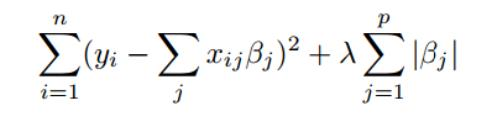# Global AI and Data Science

View Only

## Lasso Regression

####By Moloy De posted Fri June 12, 2020 12:31 AM

Lasso regression is a type of linear regression that uses shrinkage. Shrinkage is where data values are shrunk towards a central point, like the mean. The lasso procedure encourages simple, sparse models (i.e. models with fewer parameters). This particular type of regression is well-suited for models showing high levels of muticollinearity or when you want to automate certain parts of model selection, like variable selection/parameter elimination.

The acronym “LASSO” stands for Least Absolute Shrinkage and Selection Operator.

Lasso regression performs L1 regularization, which adds a penalty equal to the absolute value of the magnitude of coefficients. This type of regularization can result in sparse models with few coefficients; Some coefficients can become zero and eliminated from the model. Larger penalties result in coefficient values closer to zero, which is the ideal for producing simpler models. On the other hand, L2 regularization (e.g. Ridge regression) doesn’t result in elimination of coefficients or sparse models. This makes the Lasso far easier to interpret than the Ridge.

Lasso solutions are quadratic programming problems, which are best solved with software (like Matlab). The goal of the algorithm is to minimize:Which is the same as minimizing the sum of squares with constraint Σ |Bj|≤ s. Some of the βs are shrunk to exactly zero, resulting in a regression model that’s easier to interpret.

A tuning parameter, λ controls the strength of the L1 penalty. λ is basically the amount of shrinkage:

• When λ = 0, no parameters are eliminated. The estimate is equal to the one found with linear regression.
• As λ increases, more and more coefficients are set to zero and eliminated (theoretically, when λ = ∞, all coefficients are eliminated).
• As λ increases, bias increases.
• As λ decreases, variance increases.

If an intercept is included in the model, it is usually left unchanged.

REFERENCE : Lasso Regression: Simple Definition, a blog in Data Science Central

#GlobalAIandDataScience
#GlobalDataScience